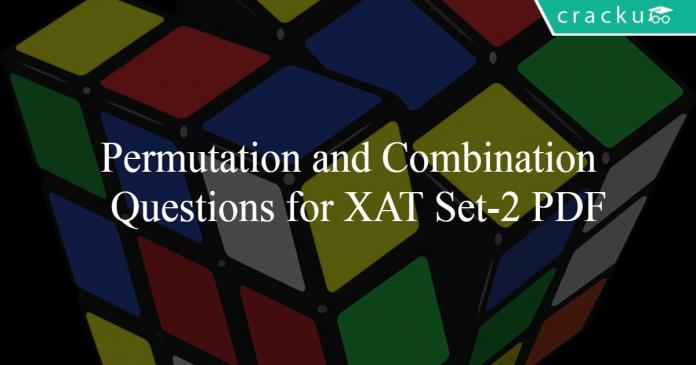# Permutation and Combination Questions for XAT Set-2 PDF

0
299Permutation and Combination Questions for XAT Set-2 PDF

# Permutation and Combination Questions for XAT Set-2 PDF

Download important Permutation and Combination Questions for XAT  Set-2 PDF based on previously asked questions in the XAT exam. Practice Permutation and Combination Questions  Set-2 PDF for the XAT exam.

InstructionsInstructions: Study the given information carefully to answer the questions that follows.

An urn contains 4 green, 5 blue, 2 red and 3 yellow marbles.

Question 1: If two marbles are drawn at random, what is the probability that both are red or at least one is red?

a) $\frac{26}{91}$

b) $\frac{1}{7}$

c) $\frac{199}{364}$

d) $\frac{133}{191}$

e) None of these

Question 2: What is the number of words formed from the letters of the word ‘JOKE’ So that the vowels and consonants alternate?

a) 4

b) 8

c) 12

d) 18

e) None of these

Instructions

Study the given information carefully and answer the question that follow:
A committee of five members is to be formed out of 3 trainees 4 professors and 6 research associates In how many different ways can this be done if____

Question 3: The committee should have all 4 professors and 1 research associate or all 3 trainees and 2 professors ?

a) 12

b) 13

c) 24

d) 52

e) None of these

Question 4: In how many different ways can the letters of the word BAKERY be arranged ?

a) 2400

b) 2005

c) 720

d) 5040

e) None of these

Instructions

Answer the following questions based on the information given below.
A bowl contains 4 red, 3 green, 2 blue and 5 black marbles.

Question 5: If four marbles are drawn at random, what is the probability that two are red and two are blue ?

a) $\frac{6}{1001}$

b) $\frac{1}{143}$

c) $\frac{1}{13}$

d) $\frac{6}{91}$

e) None of these

Question 6: In a sample , if a person is picked up randomly, the probability that the person is a smoker is $\frac{3}{5}$, and that of the person being male is $\frac{1}{2}$ .What is the probability that the person is both male as well as a smoker ?

a) $\frac{10}{11}$

b) $\frac{1}{5}$

c) $\frac{3}{5}$

d) Cannot be determined

e) None of these

Question 7: A committee of 6 members is to be selected from a group of 8 men and 6 women in such as way that at least 3 men are there in the committee. In how many different ways can it be done ?

a) 2506

b) 2534

c) 1120

d) 1050

e) None of these

Question 8: In how many different ways can the letters of the word ‘QUOTED’ be arranged

a) 720

b) 360

c) 1440

d) 320

e) None of these

Instructions

Study the given information carefully and answer the questions that follow:
An urn contains 3 red, 6 blue, 2 green and 4 yellow marbles.

Question 9: If three marbles are picked at random, what is the probability that two are blue and one is yellow ?

a) 2/15

b) 6/91

c) 12/91

d) 3/15

e) None of these

Question 10: In how many different ways can the letters of the word “PRIDE” be arranged ?

a) 60

b) 120

c) 15

d) 360

e) None of these

Answers & Solutions:

1) Answer (E)

Out of two red marbles two can be choosen in 2C2 ways
Out of two red marbles one can be choosen in 2C1 ways
Sum of blue, yellow and green marbles = 12
Out of 12 marbles one can be choosen in 12C1 ways
The probability that both are red or atleast one is red = [2C2 + (2C1 x 12C1)]/14C2 = $\frac{25}{91}$

2) Answer (B)

Word name: ‘JOKE’ Vowels: O, E
Consonants: J, K
∴ Possible arrangement beginning with consonant: JOKE, KOJE, JEKO, KEJO = 4 Numbers
beginning with vowel: OJEK, OKEJ, EJOK, EKOJ = 4 Numbers
Required number = 4+4 = 8 numbers

3) Answer (A)

4 professors and 1 resarch associate can be selected in $^4C_4*^6C_1 = 6$ ways

Similarly 3 trainees and 2 professors would be selected in $^3C_3*^4C_2$ ways = 6

Total ways = 6+6 = 12

4) Answer (C)

As there are 6 different letters in the word “Bakery” hence, total number of ways of arranging it will be = 6! = 720

5) Answer (A)

Probability of drawing two red and two blue balls = $^4C_2 * ^2C_2 / ^{14}C_4$ = 6 / 1001

Option a) is the correct answer.

6) Answer (D)

Let’s assume the sample size is 100. Let the number of male smokers be x.

Total number of smokers = 3/5 * 100 = 60

Number of men = 1/2 * 100 = 50

Hence, number of male non-smokers is 50-x. Number of female smokers is 60-x and number of female non-smokers is x-10.

Hence, probability of a person picked at random being a smoker and a male = x/100

As we do not know the value of x, we cannot determine the probability. Hence, option D.

7) Answer (B)

There are a total of 8 men and 6 women.

Number of ways in which the committee has exactly 3 men is $^8C_3 \times ^6C_3 = 1120$
Number of ways in which the committee has exactly 4 men is $^8C_4 \times ^6C_2 = 1050$
Number of ways in which the committee has exactly 5 men is $^8C_5 \times ^6C_1 = 336$
Number of ways in which the committee has exactly 6 men is $^8C_6 \times ^6C_0 = 28$

Hence, the total is $1120 + 1050 + 336 + 28 = 2534$

8) Answer (A)

Total number of arrangement= 6!=720

9) Answer (C)

Total number of marbles in the urn = 15

P(S) = Total possible outcomes

= Selecting 3 marbles at random out of 15

=> $P(S) = ^{15} C_3 = \frac{15 \times 14 \times 13}{1 \times 2 \times 3}$

= $455$

P(E) = Favorable outcomes

= Selecting 2 blue and 1 yellow marble.

=> $P(E) =C^6_2 \times C^4_1$

= $\frac{6 \times 5}{1 \times 2} \times 4$

= $60$

$\therefore$ Required probability = $\frac{P(E)}{P(S)}$

= $\frac{60}{455} = \frac{12}{91}$

10) Answer (B)

The word ‘PRIDE’ consists of 5 distinct letters

=> Number of arrangements = $5!$

= $5 \times 4 \times 3 \times 2 \times 1 = 120$

We hope this Permutation and Combination Questions Set-2 PDF for XAT with Solutions will be helpful to you.